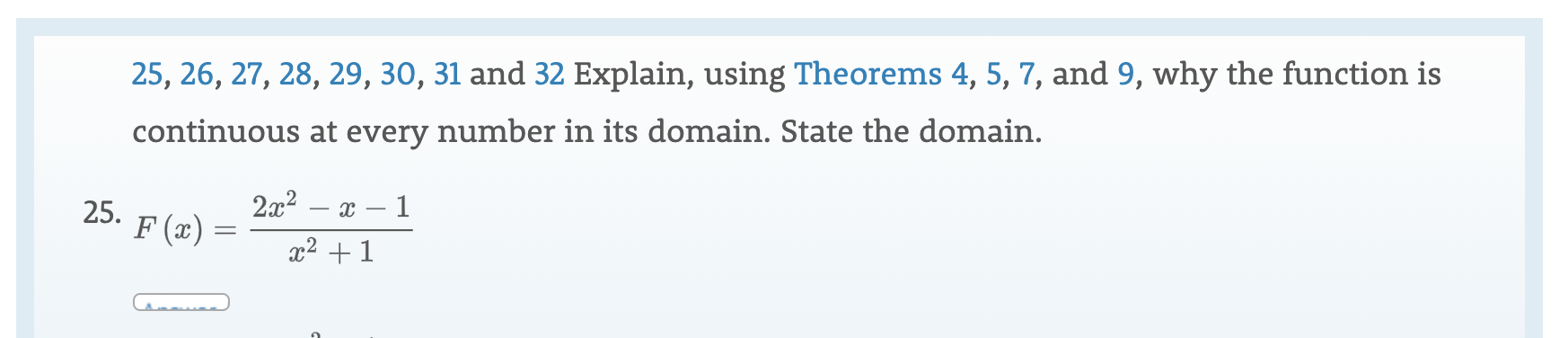# 25, 26, 27, 28, 29, 30, 31 and 32 Explain, using Theorems 4, 5, 7, and 9, why the function iscontinuous at every number in its domain. State the domain.2a225. F(x)12 1

Question
12 viewshelp_outlineImage Transcriptionclose25, 26, 27, 28, 29, 30, 31 and 32 Explain, using Theorems 4, 5, 7, and 9, why the function is continuous at every number in its domain. State the domain. 2a2 25. F(x) 1 2 1 fullscreen
check_circle

Step 1

Continuity:

A function f(x) is said to be continuous on the interval [a,b] if it is continuous at each point in the interval.

Step 2

Given that

Step 3

The given function is rational form so it is continuous on its domain.

And the domain of F(x) is every value of x for which the denominator i...

### Want to see the full answer?

See Solution

#### Want to see this answer and more?

Solutions are written by subject experts who are available 24/7. Questions are typically answered within 1 hour.*

See Solution
*Response times may vary by subject and question.
Tagged in

### Calculus